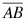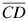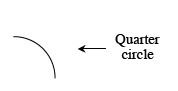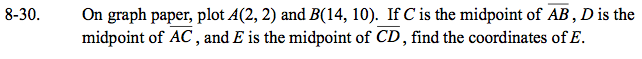Home > GC > Chapter 8 > Lesson 8.1.3 > Problem8-30

8-30.

On graph paper, plot A(2, 2) and B(14, 10). If C is the midpoint of, D is the midpoint of, and E is the midpoint of, find the coordinates of E. 8-30 HW eTool (Desmos). Homework Help ✎When finding the midpoint remember to find the average of the x-coordinates and the average of the y-coordinates for the two points.

Use the eTool to visualize the problem and solve.
Click the link at right for the full version of the eTool: GC 8-30 HW eTool